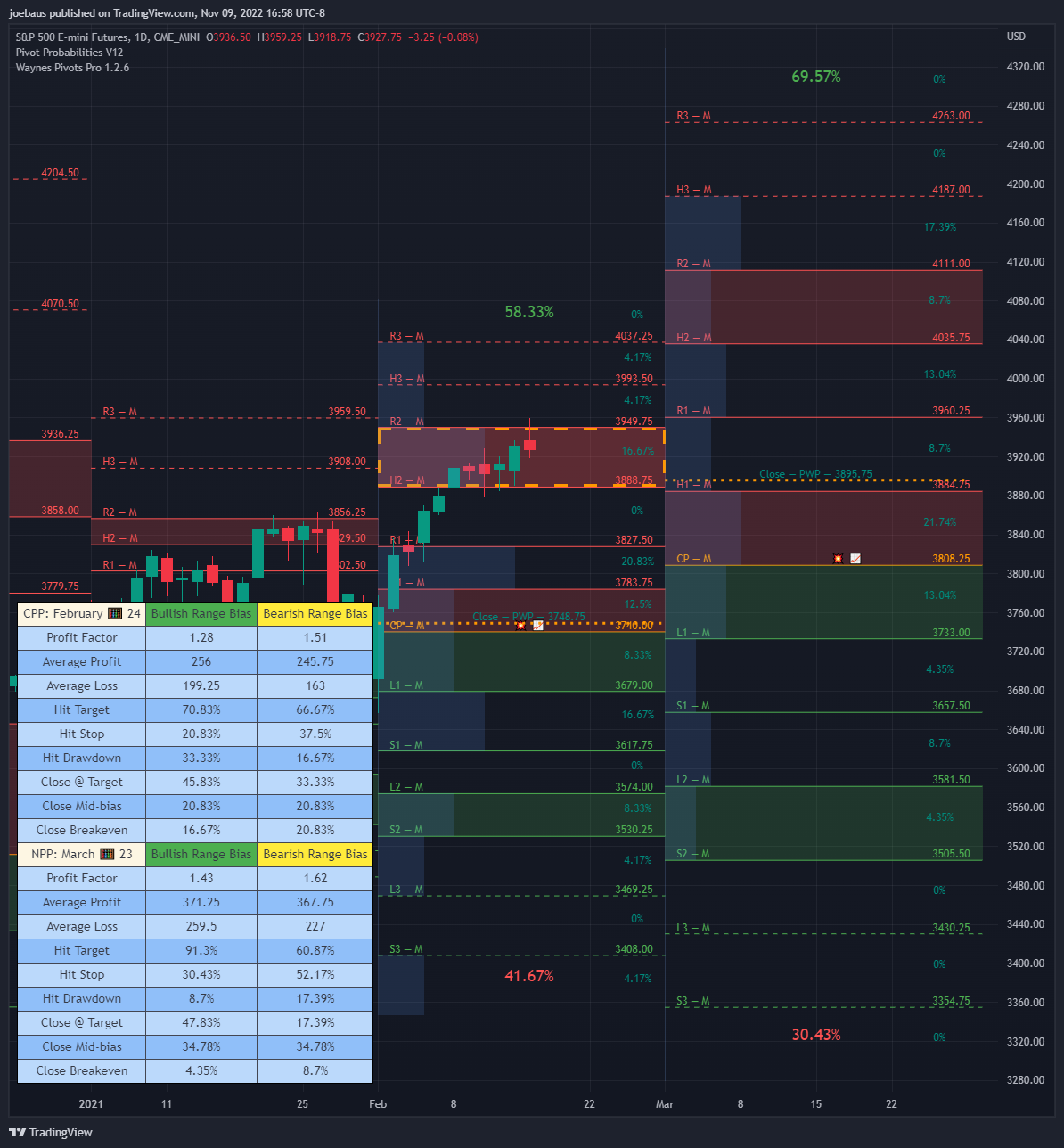Pivot Probabilities
Get historical probabilities based on seasonal price action between any two pivot points.

## Features

• Get the historical statistics of how often price moved between any two pivot points.
• Probability weighted pivot point can give an average target entry price and target exit price based on seasonality.
• Displays the next week's and month's probabilities.
• Probabilities Dashboard gives historical odds, risk/reward ratio, and win rates of pivot point trading strategies.

Use code BLACKFRI for 10% off your purchase!

What is Whop?

Note: this indicator does not draw the pivot point lines, so all purchases of Pivot Probabilities comes included with access to Wayne's Pivots Pro.

#### Basic Interpretation

Once probabilities for the current and next period (week, month) are selected, we can select what OHLC data to analyze.

Below we'll look at using High data to find likely tops.

The red boxes are the probability histogram, and the size of each box is proportional to the probability of an event occurring. The orange dotted line is the Probability Weighted Pivot (PWP). We can see January's PWP and February's. The PWP is an average, and works like the mean of the probability histogram. When using High data, we are going back in time, checking every January's highest high, finding what two pivot points it's between, and then creating the probability histogram and PWP after taking every year into account.

The Probability Dashboard can be seen to the bottom left. The green and red lit cells on the dashboard are a simple way of identifying the historical performance of a pivot point trade setup. Green means that a setup at least has 2 to 1, risk to reward odds. Red means that there is less than 1 to 1 odds. Yellow is shown when the risk to reward odds are between 1 and 2.

#### Probability Dashboard

Location on the chart, size, columns, rows, and colors of the dashboard is fully customizable. Here is a breakdown of all the statistics in the dashboard:

• Profit Factor is the theoretical return on a single unit of risk invested. A PF of 1.4 means for 1 unit of risk, there is a net 0.4 units of reward.
• Average Profit and Average Loss are conservative estimates using PWPs. Used to calculate Profit Factor.
• Hit Target odds are the respective number of historic bottoms or tops past a target pivot.
• Hit Stop odds are the respective number of historic bottoms or tops past a stop loss pivot.
• Hit Drawdown odds is your likelihood of going below the conservative pivot point entry, and not hitting your stop loss.
• Close @ Target odds sums the total number of closing probabilities above a setup's target pivot.
• Close Mid-bias odds sum the total number of closing probabilities between your setup's entry zone and target zone.
• Close Breakeven odds return the likelihood of closing between the setup's two entry zone pivots.